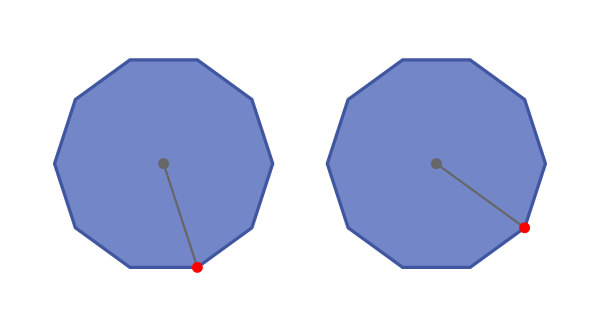# Decagons - polygons with 10 sides

By Martin McBride, 2022-10-10
Tags: decagon 10 sided shape regular polygon irregular polygon
Categories: gcse geometryA decagon is a flat shape with 10 straight sides.

## Regular and irregular decagons

A regular decagon is a 10-sided shape where every side is the same length and every corner has the same angle. All regular decagons have the same shape, like this: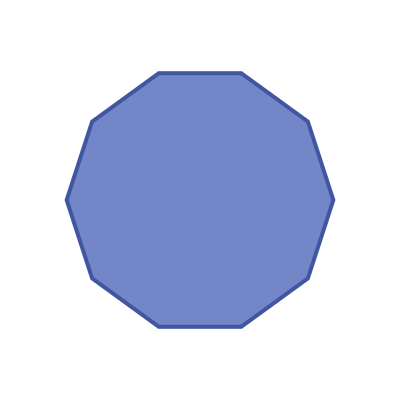An irregular decagon is a 10 sides shape where not every side and angle are equal. There are many different irregular decagon shapes, here is an example: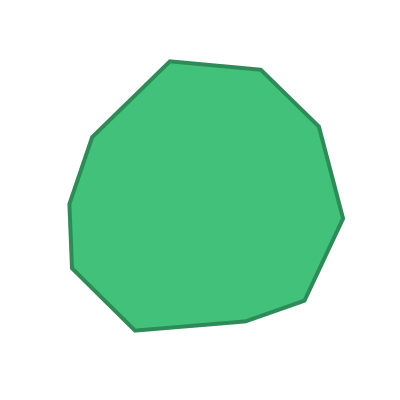## Name

The name decagon is a combination of the words deca (Greek meaning five) and gonia (Greek meaning corner).

Decagons are sometimes called 10-gons.

## Interior angles

The interior angles of a decagon are shown here: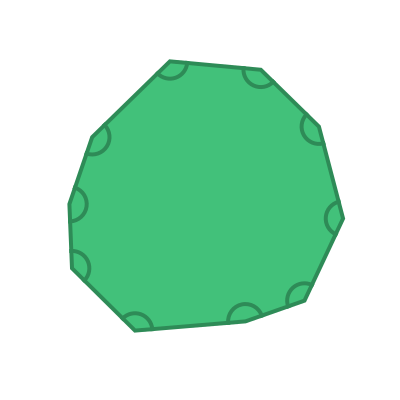The sum of these 10 angles is given by the formula:

sum of interior angles = (n - 2) x 180


Where n is the number of sides. In this case, the number of sides n is 10, so the sum of the interior angles is:

(10 - 2) x 180 = 1440 degrees


For a regular decagon, all the interior angles are equal: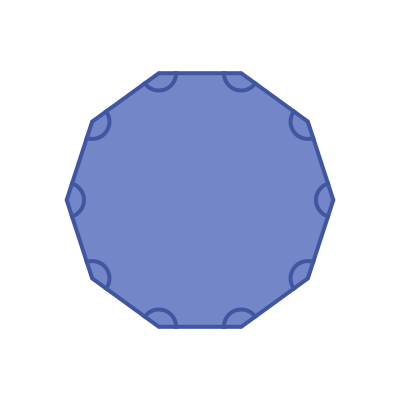This means that the interior angle of a regular decagon is:

1440 / 10 =  144 degrees


## Exterior angles

The exterior angles of a decagon are shown here: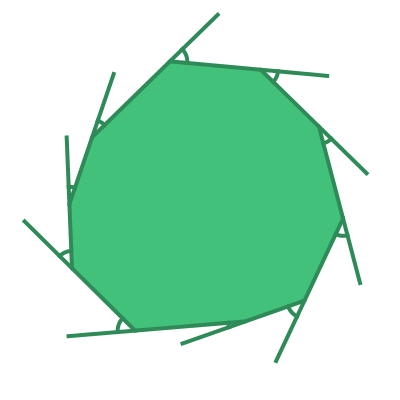The sum of the exterior angles of any polygon is 360 degrees.

For a regular decagon, all the interior angles are equal: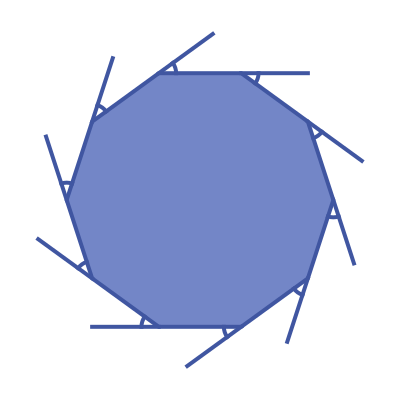This means that the exterior angle of a regular decagon is:

360 / 10 =  36 degrees


## Symmetry of regular decagons

A regular decagon has 10 lines of symmetry. This means that it can be reflected over each of the 10 grey lines shown here: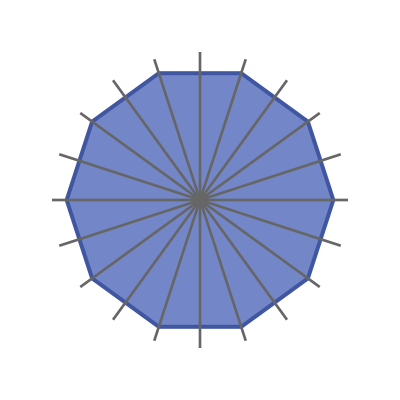A regular decagon has rotational symmetry of order 10. This means that if it rotated about its centre by a 10th of a full turn, it will map onto itself: